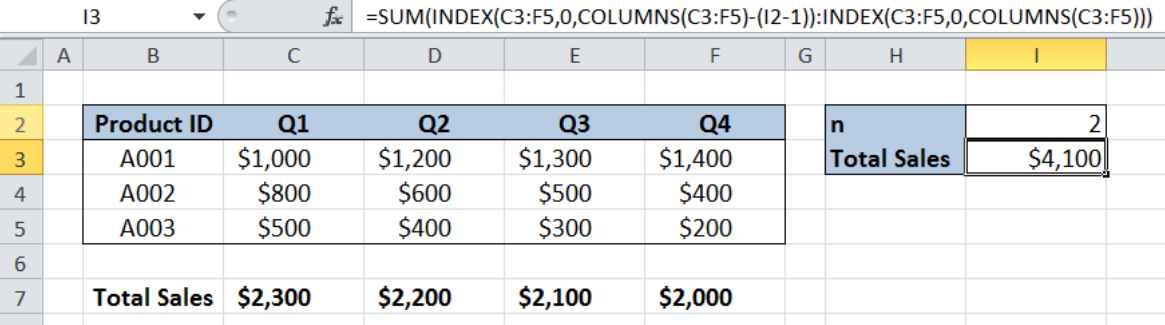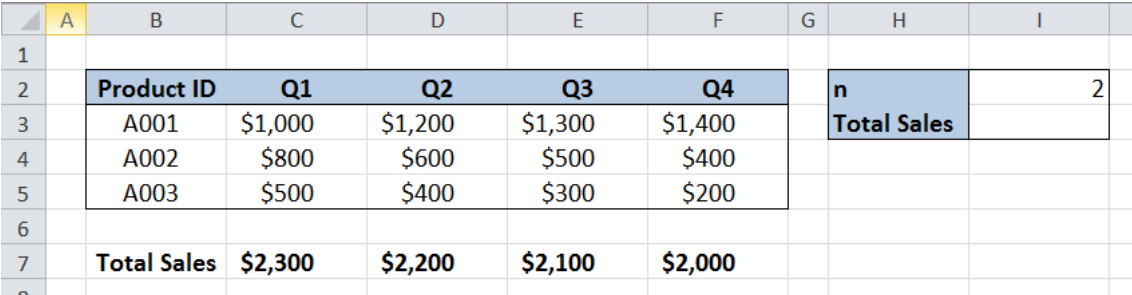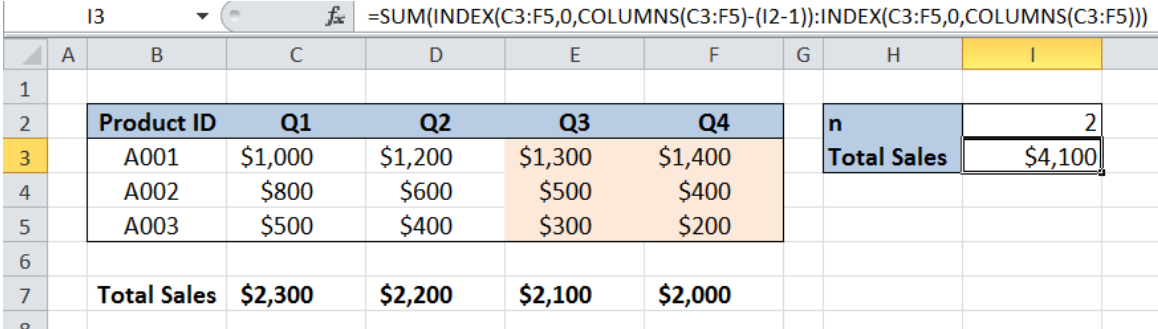Get instant live expert help with Excel or Google Sheets“My Excelchat expert helped me in less than 20 minutes, saving me what would have been 5 hours of work!”

#### Post your problem and you’ll get Expert help in seconds.

Your message must be at least 40 characters
Our professional Expert are available now. Your privacy is guaranteed.

# Sum last n columns

In Excel, we are able to sum the last n columns of any data set by using the SUM, INDEX and COLUMNS functions. The SUM function adds all given values, the INDEX function returns a value or reference to a cell, while COLUMNS returns the number of columns in an array. This step by step tutorial will assist all levels of Excel users in finding the sum of the last n columns.Figure 1. Final result: Sum last n columns

Final formula: `=SUM(INDEX(C3:F5,0,COLUMNS(C3:F5)-(I2-1)):INDEX(C3:F5,0,COLUMNS(C3:F5)))`

## Syntax of SUM Function

`=SUM(number1,[number2],...])`

• number1 – any number, array or cell reference whose values we want to add
• Only number1 is required; succeeding numbers are optional

## Syntax of INDEX function

`=INDEX(array, row_num, column_num)`

The parameters are:

• array – a range of cells where we want to retrieve some data
• row_num – the row in the array from which we want to retrieve data
• column_num – the column in the array from which we want to retrieve data

## Syntax of COLUMNS Function

`=COLUMNS(array)`

• array – reference to a range of cells whose number of columns we want to determine

## Setting up Our Data

Our table consists of Product ID (column B) and the quarterly sales in columns C, D, E and F.  Cell I2 contains the number of columns that correspond to the last n quarters we want to sum. The resulting sum will be in cell I3.Figure 2. Sample data to sum last n columns

## Sum last n columns

To sum the sales for the last two quarter, we will follow these steps:

Step 1.  Select cell I3

Step 2.  Enter the formula: `=``SUM(INDEX(C3:F5,0,COLUMNS(C3:F5)-(I2-1)):INDEX(C3:F5,0,COLUMNS(C3:F5)))`

Step 3.  Press ENTER

It is important to note that in the INDEX formula, we set the row number to zero “0” in order to lookup the entire column.Figure 3. Entering the formula to sum the last two columns

Array C3:F5 is the data range that we have.  “COLUMNS(C3:F5)” returns 4, because there are four columns in the data range.  “I2-1” returns 1 because “I2” is equal to 2.

Hence, the SUM formula is simplified and shown as an array of two INDEX formulas.

`=SUM(INDEX(C3:F5,0,3):INDEX(C3:F5,0,4))`

Finally, the formula sums the third and fourth column of the range C3:F5, resulting to \$4,100.  We have now successfully obtained the sum of the last two columns of our data set.

Most of the time, the problem you will need to solve will be more complex than a simple application of a formula or function. If you want to save hours of research and frustration, try our live Excelchat service! Our Excel Experts are available 24/7 to answer any Excel question you may have. We guarantee a connection within 30 seconds and a customized solution within 20 minutes.

### Did this post not answer your question? Get a solution from connecting with the expert.Another blog reader asked this question today on Excelchat:
Solution examplesI need a formula that will check if 2 or more cells containing names match then add the adjacent cells to matching name together.
Solved by F. D. in 59 minsI need help on index and match where the return is not correct
Solved by B. B. in 20 minsI have a database extract that is showing the same clients multiple times in the list. How can I write a formula in excel to look at the column with the client names and return only unique records on another sheet? I want to run this over several files so wanting to avoid using pivot tables which is how I would normal do this.
Solved by X. J. in 20 minsI need a formula to pop at the upper left corner of a spreadsheet. If I enter the month "January," I want the column number sum of January =SUM(AB11:AB75) from another section on the same excel page to pop right below the "January" cell, and not display the formula expression, but see the \$100.
Solved by T. Q. in 40 minsCan't add (SUM) in imported numbers from bank account
Solved by F. C. in 40 mins## Subscribe to Excelchat.coAnother blog reader asked this question today on Excelchat: